# Sensorless Field Oriented Control Applied for an Induction Machine by Using the Discontinuous PWM Strategy

Sensorless Field Oriented Control Applied for an Induction Machine by Using the Discontinuous PWM Strategy
Electromechanical Department, Institute of Mines, Echahid Cheikh Larbi Tebessi University, Tebessa 12002, Algeria

Environment Laboratory, Echahid Cheikh Larbi Tebessi University, Tebessa 12002, Algeria

LSELM Laboratory, Badji-Mokhtar University, Annaba 23000, Algeria

Corresponding Author Email:
Page:
9-14
|
DOI:
https://doi.org/10.18280/ejee.250102
10 November 2022
|
Revised:
17 December 2022
|
Accepted:
23 December 2022
|
Available online:
28 Feburary 2023
| Citation

OPEN ACCESS

Abstract:

The study presented in this paper consists in the modeling and optimization of the performance of the control of an induction motor (IM) by the application of the sensorless field oriented control (SFOC), using the strategy of the discontinuous pulse width modulation (DPWM) to switch the transistors which form the three-phase voltage source inverter (3Ph_VSI). The latter gives a reduction in the number and the switching losses compared to the space vector modulation (SVPWM). This leads to improve the performance of the IM. The proposed system has many advantages; provides for the decrease in noise, presents an increase in reliability and makes the material less expensive. The study of this control is done in order to show the efficiency and robustness of the method during variations in speed and torque.

Keywords:

induction machine, vector control, discontinuous pulse width modulation, inverter, field oriented control, space vector

1. Introduction

Electrical energy has long been used to produce mechanical energy through reversible electromechanical converters, which are electrical machines. Over time, this trend is accentuated in the industrial, tertiary and domestic sectors. Currently, it constitutes the majority 95% of the energy consumed in industry to provide motive power. Modern drive system technology increasingly demands precise and continuous control of speed, torque and position, while ensuring the highest possible stability, speed and efficiency [1, 2]. The direct current motor has satisfied some of these requirements but it is provided with brushes rubbing on the reed collector, which limits the power and the maximum speed and presents maintenance difficulties and operational interruptions [3, 4]. While electrical machinery prices have remained relatively stable, electronic and microcomputer component prices have fallen steadily, reducing the drive's share of the cost of variable speed drives.

For all these reasons, the orientation towards research leading to better exploitations of a robust actuator, is very justified, namely, the asynchronous cage motor and the synchronous motor [5, 6], which are robust and have a simple construction which limits the cost and increases the power to mass ratio. This is why AC machines are increasingly replacing DC motors in many areas, including servo motors.

With the progress of power electronics, linked to the appearance of fast switching components, as well as the development of control techniques [7, 8], wired or programmed, it is now possible to choose a much more advanced control structure. The FOC of asynchronous and synchronous machines can now highlight control principles making it possible to achieve performance equivalent to that of the DC machine .

This paper describes a study of the sensorless field oriented control SFOC applied to the three phase induction motor IM, using the strategy of the discontinuous pulse width modulation DPWM to switch the transistors which form the three-phase voltage source inverter 3Ph_VSI. This control technique has undergone significant development in recent years, particularly with the evolution of the integration of PWM modulation techniques such as inverter control strategies. The validity and results are verified by Simulink/MATLAB simulation.

2. System Description

The circuit consist two parts, the first contains the power part, represented by: a three-phase diode rectifier, LC filter, a three-phase inverter and the induction motor. While the second contains the control part, represented by the SFOC control and the DPWM strategy. The system description as shown in Figure 1.

## image002.png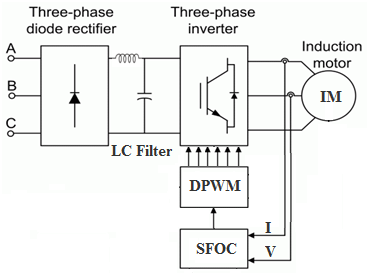Figure 1. System description

2.1 Modeling of three-phase diode rectifier

A rectifier, also called AC/DC converter or GRAETZ bridge, is a converter intended to supply a load which needs to be supplied by a voltage and a current that are both as continuous as possible, from an AC voltage source. The power supply is, most of the time [7-9], a voltage generator. The average voltage across the load is given by the following equations:

$\mathrm{U}_{\mathrm{AV}}=\frac{3 \sqrt{6}}{\pi} \mathrm{U}_{\mathrm{RMS}, \mathrm{S}}=2.33 \mathrm{U}_{\mathrm{RMS}, \mathrm{S}}$    (1)

with, URMS, S: is the RMS voltage of the AC source.

2.2 Stabilization LC filter

To correct the DC voltage source, a capacitor C is inserted at the output of the rectifier, this absorbs the difference between the different currents, and suppresses the sudden variations of the switching voltage, on the other hand, to reduce the ripple of the current and protect the inverter against the critical speed of current growth, a smoothing inductance L of internal resistance R is placed in series .

The transfer function of the LC filter is represented by the following equation:

$F(s)=\frac{1}{L C s^2+R C s+1}$    (2)

L: is the inductance in series with the rectifier.

C: is the capacitor in parallel with the rectifier.

R: is an internal resistance.

2.3 Modeling of VSI

## image005.png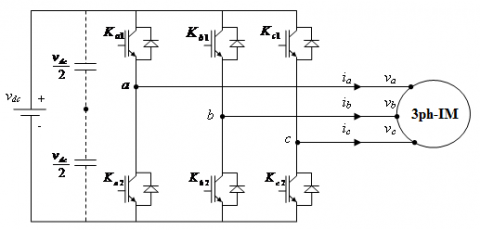Figure 2. Three-phase VSI

The VSI shown in Figure 2, delivers two levels of voltages or depending on the connection function Sx1 as shown in the Eq. (3) [11-13]:

{{\nu }_{xo}}=\left\{ \begin{align} & \frac{{{\nu }_{dc}}}{2}si{{S}_{x1}}=1\quad and{{S}_{x2}}=0 \\ & -\frac{{{\nu }_{dc}}}{2}si{{S}_{x1}}=0\quad and{{S}_{x2}}=1 \\ \end{align} \right.    (3)

where,vxo is the phase-to-neutral voltage between phase x and midpoint o.

The three phase-midpoint voltages vao, vbo and vco are expressed by:

$\left[\begin{array}{l}v_{a o} \\ v_{b o} \\ v_{c o}\end{array}\right]=\frac{v_{d c}}{2}\left[\begin{array}{l}2 S_{a 1}-1 \\ 2 S_{b 1}-1 \\ 2 S_{c 1}-1\end{array}\right]$    (4)

And the three phase-to-neutral voltages va, vb and vc are expressed by the following equation :

\left[ \begin{align} & {{v}_{a}} \\ & {{v}_{b}} \\ & {{v}_{c}} \\ \end{align} \right]=\frac{1}{3}{{v}_{dc}}\left[ \begin{matrix} 2 & -1 & -1 \\ -1 & 2 & -1 \\ -1 & -1 & 2 \\ \end{matrix} \right]\left[ \begin{matrix} {{S}_{a1}} \\ {{S}_{b1}} \\ {{S}_{c1}} \\ \end{matrix} \right]     (5)

For the generation of logic signals, there are several control techniques such as the PWM strategy.

2.3.1 Discontinuous PWM strategy

The three-phase VSI is a converter with eight switches which requires a good PWM control strategy [15, 16]. In this work, we have chosen the DPWM strategy which offers the possibility of reducing the number of switching in the inverter. The basic principle of this technique is to inject the zero voltage sequence (ZVS) to the sinusoidal reference waves, with the aim of blocking each phase for 60 degrees in two times compared to the modulation period which is 360 degrees , to generate a balanced three-phase system. Figure 3 shows the principle of this strategy.

The DPWM strategy uses the obtained modulators from the sinusoidal references by adding the ZVS equal to :

ZVS $=\operatorname{sign}\left(V_m\right) * E / 2-V_m$    (6)

where Vm is the voltage resulting from the maximum amplitude test (absolute maximum of the three reference voltages between varef, vbref and vcref).

## pi_zhu_2023-04-13_130323.png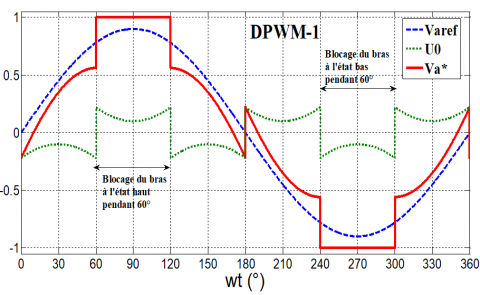Figure 3. Principle of the DPWM strategy

2.4 Sensorless field oriented control SFOC

2.4.1 Modeling of induction motor IM

IM depends on the rotational speed of the rotor. If the latter is slightly greater than that of the magnetic field of the stator, it then develops an electromagnetic force similar to that obtained with a synchronous generator [19, 20]. On the other hand, the machine does not generate its own excitation energy. For that, it will be necessary to bring this energy either by a battery of capacitors, or by a static converter control, which will stabilize its output voltage and frequency through capacitors connected across the stator. The model of induction machine IM is composed of an electrical phase windings and a rotor cage squirrel .

\left\{ \begin{align} & {{\nu }_{ds}}={{R}_{s}}{{i}_{ds}}\frac{d}{dt}{{\varphi }_{ds}}-\omega {{\varphi }_{qs}} \\ & {{\nu }_{qs}}={{R}_{s}}{{i}_{qs}}\frac{d}{dt}{{\varphi }_{qs}}-\omega {{\varphi }_{ds}} \\ & {{\nu }_{dr}}={{R}_{r}}{{i}_{dr}}\frac{d}{dt}{{\varphi }_{dr}}-\left( {{\omega }_{s}}-{{\omega }_{r}} \right){{\varphi }_{qr}} \\ & {{\nu }_{qr}}={{R}_{r}}{{i}_{qr}}\frac{d}{dt}{{\varphi }_{qr}}+\left( {{\omega }_{s}}-{{\omega }_{r}} \right){{\varphi }_{dr}} \\ \end{align} \right.    (7)

The expression for the stator and rotor flux linkages:

\left\{ \begin{align} & {{\varphi }_{ds}}={{L}_{s}}{{i}_{ds}}+{{L}_{m}}\left( {{i}_{ds}} \right.+\left. {{i}_{dr}} \right) \\ & {{\varphi }_{qs}}={{L}_{s}}{{i}_{qs}}+{{L}_{m}}\left( {{i}_{qs}} \right.+\left. {{i}_{qr}} \right) \\ & {{\varphi }_{dr}}={{L}_{r}}{{i}_{dr}}+{{L}_{m}}\left( {{i}_{ds}} \right.+\left. {{i}_{dr}} \right) \\ & {{\varphi }_{qr}}={{L}_{r}}{{i}_{qr}}+{{L}_{m}}\left( {{i}_{qs}} \right.+\left. {{i}_{qr}} \right) \\ \end{align} \right.    (8)

The electrical model is completed by this mechanical equation :

$\mathop{T}_{em}^{{}}-\mathop{T}_{r}^{{}}=j\frac{d{{\Omega }_{mec}}}{dt}+{{k}_{f}}{{\Omega }_{mec}}$    (9)

The electromagnetic torque expression as a function of stator currents and rotor flux as follows:

$T_{e m}=p \frac{L_m}{L_m+L_r}\left[i_{q s} \varphi_{d r}-i_{d s} \varphi_{q r}\right]$    (10)

2.4.2 Modeling of sensorless field oriented control of IM

The main objective of the vector control induction motors is, as in DC machines, to independently control the torque and the flux. In this order we propose to study the SFOC of the IM . The control strategy used consists to maintain the quadrate component of the flux null (φqr=0) and the direct flux equals to the reference (φdr= φr*) [3, 4]: φr* and the torque T*em as well as

$\left\{\begin{array}{c}\varphi_{d r}=\varphi_r^* \\ \varphi_{q r}=0 \\ \frac{d}{d t} \varphi_r^*=0\end{array}\right.$    (11)

Substituting (11) into (7) yields:

\left\{ \begin{align} & {{i}_{dr}}=0 \\ & {{i}_{qr}}=-\frac{\mathop{\omega }_{gl}^{*}\ \mathop{\varphi }_{r}^{*}}{\mathop{R}_{r}} \\ \end{align} \right.    (12)

with $\omega_{g l}^*=\omega_s^*-\omega_s$ ($\omega_g^* l$ is the slip speed).

After calculation and rearrangement of the electromagnetic torque and stator voltages equations, following expressions are obtained .

\left\{ \begin{align} & \mathop{i}_{dr}^{{}}=\frac{\mathop{\varphi }_{r}^{*}}{{{L}_{m}}+{{L}_{r}}}-\frac{{{L}_{m}}}{{{L}_{m}}+{{L}_{r}}}\mathop{i}_{ds}^{{}} \\ & \mathop{i}_{qr}^{{}}=\frac{{{L}_{m}}}{{{L}_{m}}+{{L}_{r}}}\mathop{i}_{qs}^{{}} \\ \end{align} \right.    (13)

with:

idr and iqr: are the rotor (d, q) axis current.

ids and iqs: are the stator (d, q) axis current.

Lr: rotor inductance.

Ls: stator inductance.

Lm: mulual inductance.

Substitution (13) into (12), obtain

$\mathop{\omega }_{gl}^{*}=\frac{\mathop{R}_{r}\ {{L}_{m}}\mathop{i}_{qs}^{{}}}{({{L}_{m}}+{{L}_{r}})}\mathop{\varphi }_{r}^{*}$    (14)

The final expression of the torque electromagnetic is

$\mathop{T}_{em}^{*}=p\frac{{{L}_{m}}}{({{L}_{m}}+{{L}_{r}})}\mathop{\mathop{i}_{qs}^{{}}\,\varphi }_{r}^{*}$    (15)

with taking into the rotor field orientation, the stator voltage Eq. (7) can be rewritten as 

$\left\{ \begin{array}{*{35}{l}} v_{ds}^{*}={{R}_{s}}{{i}_{ds}}+{{L}_{s}}\frac{d}{dt}{{i}_{ds}}-\omega _{S}^{*}\left( {{L}_{s}}{{i}_{qs}}+{{\tau }_{r}}\varphi _{r}^{*}\left( \omega _{s}^{*}-\omega _{r}^{*} \right) \right) \\ v_{qs}^{*}={{R}_{s}}{{i}_{qs}}+{{L}_{s}}\frac{d}{dt}{{i}_{qs}}+\omega _{s}^{*}\left( {{L}_{s}}{{i}_{ds}}+\varphi _{r}^{*} \right) \\ \end{array} \right.$    (16)

where, $\tau_r=\frac{L_r}{R_r}$.

Consequently, the electrical and mechanical equations for the system after these transformations in the space control may be written as follows [5, 6]:

\left\{ \begin{align} & \frac{d}{dt}{{i}_{ds}}=\frac{1}{{{L}_{s}}}\left( \nu _{ds}^{*}- \right.{{R}_{s}}{{i}_{ds}}+\mathop{\omega }_{s}^{*}\left( {{L}_{s}}{{i}_{qs}}+{{\tau }_{r}}\mathop{\varphi }_{r}^{*} \right.\left( \mathop{\omega }_{s}^{*}-\mathop{\omega }_{r}^{*})) \right. \\ & \frac{d}{dt}{{i}_{qs}}=\frac{1}{\mathop{L}_{s}}\left( \nu _{qs}^{*}- \right.{{R}_{s}}{{i}_{qs}}-\mathop{\omega }_{s}^{*}\left( \mathop{L}_{s}{{i}_{ds}}+\mathop{\varphi }_{r}^{*} \right) \\ \end{align} \right.    (17)

$\frac{d}{dt}{{\varphi }_{r}}=-\frac{{{R}_{r}}}{{{L}_{r}}+{{L}_{m}}}\left( {{\varphi }_{r}}+{{L}_{m}}{{i}_{ds}} \right)$    (18)

$\frac{d}{dt}{{\Omega }_{mec}}=\frac{1}{j}\left( \frac{p{{L}_{m}}{{i}_{qs}}\mathop{\varphi }_{r}^{*}}{({{L}_{r}}+{{L}_{m}})}-{{T}_{g}}-f{{\Omega }_{mec}} \right)$    (19)

## image015.png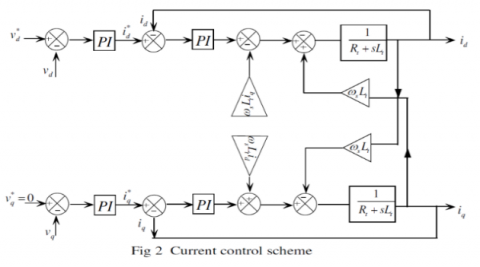Figure 4. Current control scheme

For perfect decoupling, stator current control loops (ids, iqs) are added and at their outputs the voltage (vds, vqs) are obtained. The block control diagram is shown in Figure 4 . Dynamic equation for the dc bus voltage, is derived as follows. Equating currents at the dc bus voltage, following equation is obtained:

$\mathop{i}_{dc}=\mathop{C}_{dc}\frac{d}{dt}\mathop{v}_{dc}$    (20)

The current control scheme is given in Figure 4. The references $i_d^*$  and $i_q^*$ are the outputs of the PI regulator .

\left\{ \begin{align} & \mathop{i}_{d}^{*}=\left( PI \right)\ \left( \mathop{v}_{d}^{*}-\mathop{v}_{d}^{{}} \right) \\ & \mathop{i}_{q}^{*}=\left( PI \right)\ \left( \mathop{v}_{q}^{*}-\mathop{v}_{q}^{{}} \right) \\ \end{align} \right.     (21)

3. Simulation Results

The overall system to be studied consists of a IM connected to a rectifier/inverter and controlled according to the control strategy presented above. In what follows, two simulation tests are performed to analyze the performance of the control: The first test is dedicated to the control behavior at fixed speed control followed by a load disturbance; the second is intended for the study of the control with variable speed. Using Matlab/Simulink, we verify the electrical, magnetic and mechanical quantities of the induction machine. We used the different parameters which are shown in the Table 1.

Table 1. Induction motor parameters 

 motor parameters Values P 1.5 Kw VAB 380 V frequency 50 Hz In 3.6 A N 1400 rpm Rs 4.85Ω Rr 3.085 Ω Ls 0.274H Lr 0.274H M 0.258 H f 0.00114Nm.rad-1s-1 j 0.031 Kg.m2 Tr 3N.m

To test the robustness of the regulation, we simulated unloaded starting mode for a reference speed at (100 rad/s), then the response to a load torque step (Tr=3N.m) applied at time t=1s.The simulation results are represented as follows: Figure 5 shows the stator current in the (d, q) axis. We see that there is only one ripple in the current during the transient state, representing the starting current.

Figure 6 shows the magnetic flux of the machine. We note that the component of the flux on the quadratic axis is zero during the simulation time, which represents a perfect decoupling.

Figure 7 and 8 show the angular speed of rotation and the electromagnetic torque of the machine respectively.

## tu_pian_1.jpg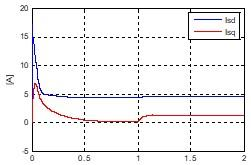Figure 5. Stator current of IM

## tu_pian_2.jpg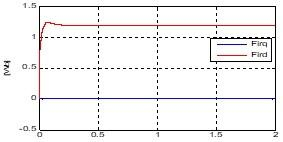Time [s]

Figure 6. Magnetic flux of IM

## tu_pian_3.jpg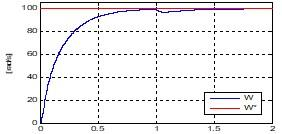Time [s]

(a)

## tu_pian_4.jpg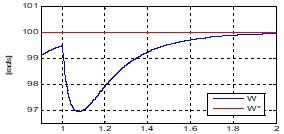Time [s]

(b)

Figure 7. Speed of IM, a) Speed and its reference and b) Speed zoom

## tu_pian_5.jpg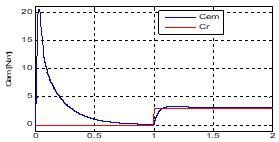Time [s]

Figure 8. Electromagnetic torque and its reference of IM

These results show the decoupling between the electromagnetic torque and the rotor flux expressed by the response of the isd and isq components of the stator current. During the load step, the torque perfectly follows the reference with an influence on the speed which then reaches its reference value (fast disturbance rejection).

3.2 Response to speed reversal

We simulated the system for a change in the speed setpoint from +100 to -100 rad/s, from time t=0.7 s, and a second inversion at a speed of +30 rad/s from time t=1.4 s.

## tu_pian_6.png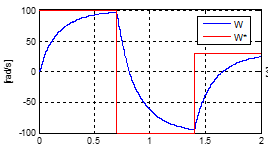Time [s]

Figure 9. Speed and its reference of IM

## tu_pian_7.png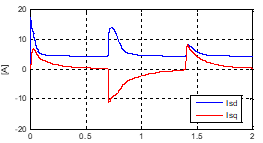Time [s]

Figure 10. Stator current of IM

## tu_pian_8.png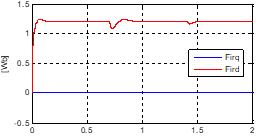Time [s]

Figure 11. Magnetic flux of IM

## tu_pian_9.png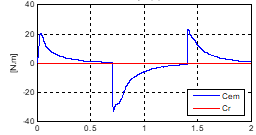Time [s]

Figure 12. Electromagnetic torque and its reference of IM

We notice in the Figures 5-12, the speed is obtained without overshoot despite the dynamics of the flow. This shows that the analytical approach proposed for the use of the discontinuous PWM technique is quite rigorous. During start-up, we also observe an overshoot of the torque due to the initialization of the flux.

4. Conclusion

In this paper, the subject consists of improving the performance of an induction motor based on sensorless field-oriented control using the discontinuous PWM strategy. The description of the latter is presented, adopting a mathematical model that defines the different equations describing the operation of the machine.

The simulation results ensure good dynamic control of the electromagnetic torque and speed of IM with very small changes around its reference value. And finally, we can say that the discontinuous PWM strategy adapts well the vector control of the alternative machines and gives many advantages with less power losses and a great dynamic stability.

References

 Dadabaev, S.T., Gracheva, E.I., Dadabaeva, Z.A. (2021). Study of starting transition processes of asynchronous motor at a lowered mains voltage frequency. In Sustainable Energy Systems: Innovative Perspectives: SES 2020, 141: 206-213. https://doi.org/10.1007/978-3-030-67654-4_23

 Elgbaily, M., Anayi, F., Alshbib, M.M. (2022). A combined control scheme of direct torque control and field-oriented control algorithms for three-phase induction motor: Experimental validation. Mathematics, 10(20): 3842. https://doi.org/10.3390/math10203842

 Englert, T., Graichen, K. (2020). Nonlinear model predictive torque control and setpoint computation of induction machines for high performance applications. Control Engineering Practice, 99: 104415. https://doi.org/10.1016/j.conengprac.2020.104415

 Azzoug, Y., Sahraoui, M., Pusca, R., Ameid, T., Romary, R., Cardoso, A.J.M. (2021). High-performance vector control without AC phase current sensors for induction motor drives: Simulation and real-time implementation. ISA Transactions, 109: 295-306. https://doi.org/10.1016/j.isatra.2020.09.021

 Sun, X., Wu, M., Lei, G., Guo, Y., Zhu, J. (2020). An improved model predictive current control for PMSM drives based on current track circle. IEEE Transactions on Industrial Electronics, 68(5): 3782-3793. https://doi.org/10.1109/TIE.2020.2984433

 Xu, Y., Li, S., Zou, J. (2021). Integral sliding mode control based deadbeat predictive current control for PMSM drives with disturbance rejection. IEEE Transactions on Power Electronics, 37(3): 2845-2856. https://doi.org/10.1109/TPEL.2021.3115875

 Premkumar, K., Kandasamy, P., Vishnu Priya, M., Thamizhselvan, T., Ron Carter, S.B. (2020). Three-phase rectifier control techniques: A comprehensive literature survey. International Journal of Scientific & Technology Research, 9(01): 3183-3188.

 Ramasamy, P., Krishnasamy, V. (2020). SVPWM control strategy for a three phase five level dual inverter fed open-end winding induction motor. ISA Transactions, 102, 105-116. https://doi.org/10.1016/j.isatra.2020.02.034

 Zhang, Y., Wang, Z., Jiao, J., Liu, J. (2019). Grid-voltage sensorless model predictive control of three-phase PWM rectifier under unbalanced and distorted grid voltages. IEEE Transactions on Power Electronics, 35(8): 8663-8672. https://doi.org/10.1109/TPEL.2019.2963206

 Salehi, A., Moloudian, G., Setoudeh, F. (2019). Design, simulation and manufacturing microstrip low-pass filter by wide stopband and changing fast situation from passing state to stopping. IETE Journal of Research, 65(4): 487-493. https://doi.org/10.1080/03772063.2018.1433079

 Debnath, T., Resalayyan, R., Imthias, M., Gopakumar, K., Umanand, L., Jarzyna, W. (2022). A multilevel inverter for instantaneous voltage balancing of single sourced stacked dc-link capacitors for an induction motor load. IEEE Transactions on Power Electronics, 37(9): 10633-10641. https://doi.org/10.1109/TPEL.2022.3163725

 Gupta, S.K., Khan, M.A. (2020). Space vector modulation strategy for three level operation of five-phase two-level dual voltage source inverter system. In 2020 IEEE 17th India Council International Conference (INDICON), New Delhi, India, pp. 1-4. https://doi.org/10.1109/INDICON49873.2020.9342205

 Dhanamjayulu, C., Khasim, S.R., Padmanaban, S., Arunkumar, G., Holm-Nielsen, J.B., Blaabjerg, F. (2020). Design and implementation of multilevel inverters for fuel cell energy conversion system. IEEE Access, 8: 183690-183707. https://doi.org/10.1109/ACCESS.2020.3029153

 Mishra, P., Maheshwari, R. (2019). Design, analysis, and impacts of sinusoidal LC filter on pulsewidth modulated inverter fed-induction motor drive. IEEE Transactions on Industrial Electronics, 67(4): 2678-2688. https://doi.org/10.1109/TIE.2019.2913824

 Patel, H., Chandwani, H. (2021). Simulation and experimental verification of modified sinusoidal pulse width modulation technique for torque ripple attenuation in Brushless DC motor drive. Engineering Science and Technology, an International Journal, 24(3): 671681. https://doi.org/10.1016/j.jestch.2020.11.003

 Kim, S.M., Lee, K.B. (2019). A modified third harmonic pulse-width modulation for reduced switching loss in cascaded H-bridge multilevel inverters. IFAC-PapersOnLine, 52(4): 472-476. https://doi.org/10.1016/j.ifacol.2019.08.255

  Zaamouche, F., Saad, S., Hamiche, L. (2020). Discontinuous PWM applied for a three-phase five-level CHB inverter fed by PV solar-boost converter. European Journal of Electrical Engineering, 22(2): 153-161. https://doi.org/10.18280/ejee.220209

 Houam, A., Zaamouche, F., Ounnas, D. (2022). DPWM applying for five-level npc vsi powered by PV-boost converter based on takagi sugeno fuzzy model. European Journal of Electrical Engineering, 24(2): 105-112. https://doi.org/10.18280/ejee.240205

 Belkacemi, B., Saad, S., Ghemari, Z., Zaamouche, F., Khazzane, A. (2020). Detection of induction motor improper bearing lubrication by discrete wavelet transforms (DWT) decomposition. Instrumentation Mesure Métrologie, 19(5): 347-354. https://doi.org/10.18280/i2m.190504

 El Daoudi, S., Lazrak, L., El Ouanjli, N., Lafkih, M.A. (2021). Sensorless fuzzy direct torque control of induction motor with sliding mode speed controller. Computers & Electrical Engineering, 96, 107490 https://doi.org/10.1016/j.compeleceng.2021.107490

 El Ouanjli, N., Motahhir, S., Derouich, A., El Ghzizal, A., Chebabhi, A., Taoussi, M. (2019). Improved DTC strategy of doubly fed induction motor using fuzzy logic controller. Energy Reports, 5, 271-279. https://doi.org/10.1016/j.egyr.2019.02.001

 Ammar, A. (2019). Performance improvement of direct torque control for induction motor drive via fuzzy logic-feedback linearization: Simulation and experimental assessment. COMPEL-The International Journal for Computation and Mathematics in Electrical and Electronic Engineering, 38(2): 672-692.

 Elgbaily, M., Anayi, F., Packianather, M. (2022). Genetic and particle swarm optimization algorithms based direct torque control for torque ripple attenuation of induction motor. Materials Today: Proceedings, 67: 577-590. https://doi.org/10.1016/j.matpr.2022.08.293

 H Magdy, M., Abu-Zaid, S., Elwany, M.A. (2021). Artificial intelligent techniques based on direct torque control of induction machines. International Journal of Power Electronics and Drive Systems, 12(4): 2070. https://doi.org/10.11591/ijpeds.v12.i4.pp2070-2082